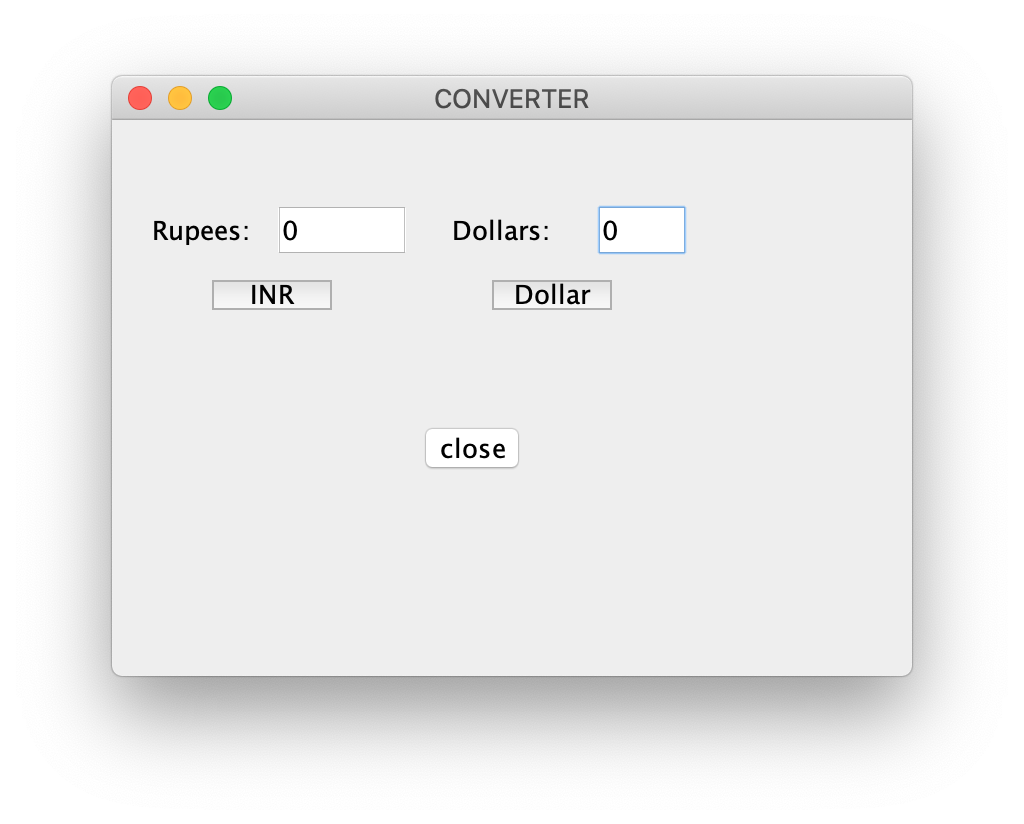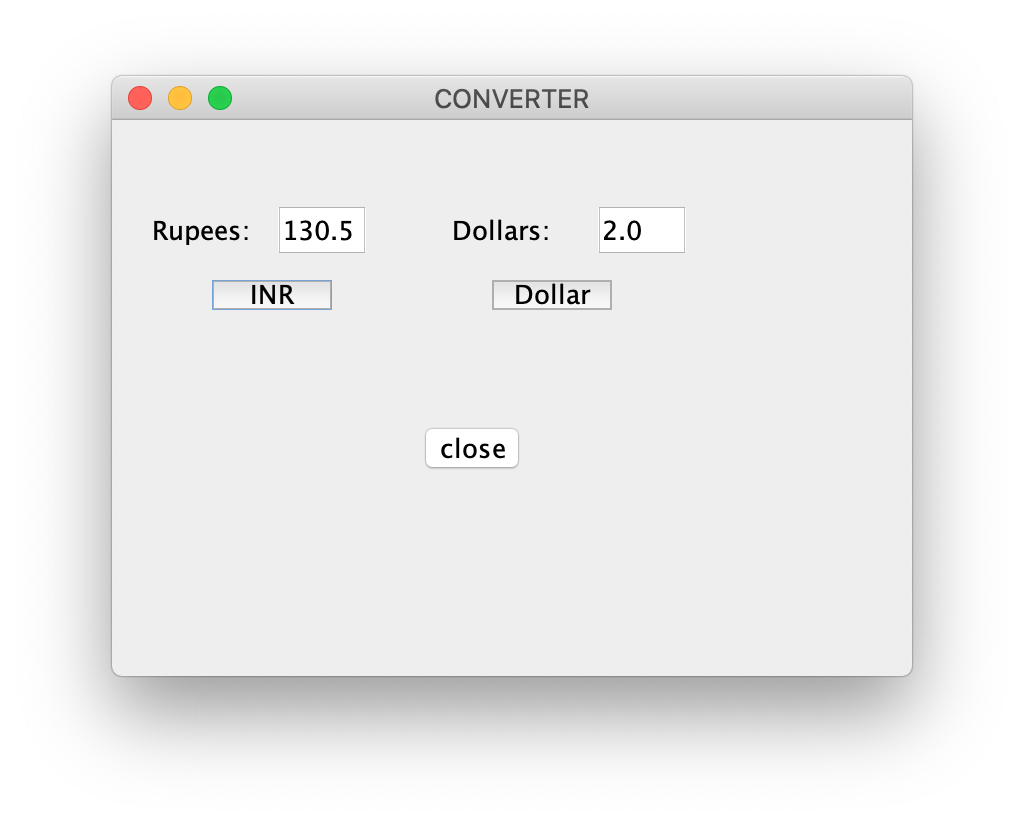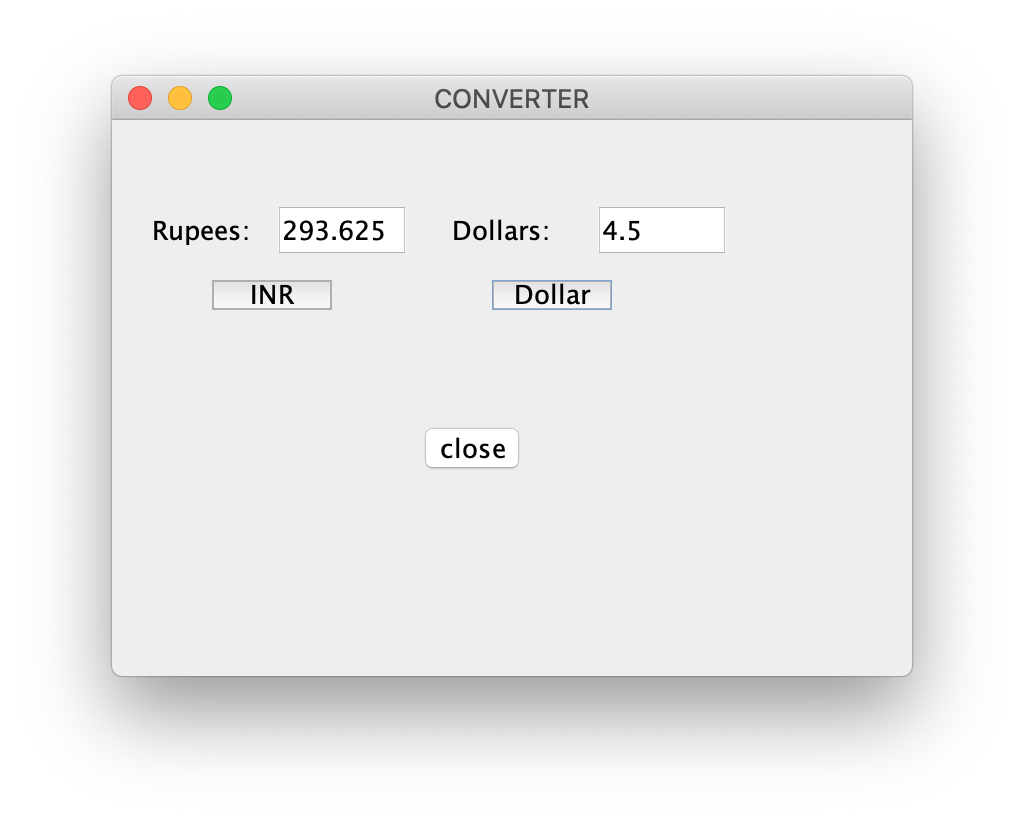Related Articles

# Java program to convert Currency using AWT

• Difficulty Level : Easy
• Last Updated : 08 May, 2020

Swing is a part of the JFC (Java Foundation Classes). Building Graphical User Interface in Java requires the use of Swings. Swing Framework contains a large set of components which allow a high level of customization and provide rich functionalities, and is used to create window-based applications. Java swing components are lightweight, platform-independent, provide powerful components like tables, scroll panels, buttons, list, colour chooser, etc.

In this article, we’ll see how to make a currency converter which includes conversion between INR and Dollar. Two text fields are implemented with the labels Rupees and Dollar.

Note: It is assumed that 1 dollar is equal to 65.25 rupees.

Examples:

Input: INR = 130.5
Output: 2.0
Explanation:
One dollar is 65.25 rupees. So, 130.5 rupees is two dollars.

Input: Dollar = 4.5
Output: 293.625

## Recommended: Please try your approach on {IDE} first, before moving on to the solution.

Approach: To solve this problem, the following steps are followed:

1. First, we need to create a frame using JFrame.
2. Then, create two labels, two textfields and three buttons(the first button for rupees and the second button is for the dollar) using JLabel, JTextField and JButton.
3. Name these components accordingly and set their bounds.
4. Now, in order to perform the conversion on button click, we need to add Event Handlers. In this case, we will add ActionListener to perform an action method known as actionPerformed in which first we need to get the values from the text fields which is default as a “string”.
5. So, in order to perform mathematical operations, we need to convert them into double data type using Double.parseDouble(Object.getText()) and again converting from double to string to place the final value in the other text field using String.valueOf(object).
6. Finally, for changing the values, we use Object.setText(object), the second object is for selecting which field we want to replace.

Below is the implementation of the above approach:

 `// Java program to convert from``// rupee to the dollar and vice-versa``// using Java Swing`` ` `import` `javax.swing.*;``import` `java.awt.*;``import` `java.awt.event.*;``public` `class` `GFG {`` ` `    ``// Function to convert from rupee``    ``// to the dollar and vice-versa``    ``// using Java Swing``    ``public` `static` `void` `converter()``    ``{`` ` `        ``// Creating a new frame using JFrame``        ``JFrame f = ``new` `JFrame(``"CONVERTER"``);`` ` `        ``// Creating two labels``        ``JLabel l1, l2;`` ` `        ``// Creating two text fields.``        ``// One for rupee and one for``        ``// the dollar``        ``JTextField t1, t2;`` ` `        ``// Creating three buttons``        ``JButton b1, b2, b3;`` ` `        ``// Naming the labels and setting``        ``// the bounds for the labels``        ``l1 = ``new` `JLabel(``"Rupees:"``);``        ``l1.setBounds(``20``, ``40``, ``60``, ``30``);``        ``l2 = ``new` `JLabel(``"Dollars:"``);``        ``l2.setBounds(``170``, ``40``, ``60``, ``30``);`` ` `        ``// Initializing the text fields with``        ``// 0 by default and setting the``        ``// bounds for the text fields``        ``t1 = ``new` `JTextField(``"0"``);``        ``t1.setBounds(``80``, ``40``, ``50``, ``30``);``        ``t2 = ``new` `JTextField(``"0"``);``        ``t2.setBounds(``240``, ``40``, ``50``, ``30``);`` ` `        ``// Creating a button for INR,``        ``// one button for the dollar``        ``// and one button to close``        ``// and setting the bounds``        ``b1 = ``new` `JButton(``"INR"``);``        ``b1.setBounds(``50``, ``80``, ``60``, ``15``);``        ``b2 = ``new` `JButton(``"Dollar"``);``        ``b2.setBounds(``190``, ``80``, ``60``, ``15``);``        ``b3 = ``new` `JButton(``"close"``);``        ``b3.setBounds(``150``, ``150``, ``60``, ``30``);`` ` `        ``// Adding action listener``        ``b1.addActionListener(``new` `ActionListener() {``            ``public` `void` `actionPerformed(ActionEvent e)``            ``{``                ``// Converting to double``                ``double` `d``                    ``= Double.parseDouble(t1.getText());`` ` `                ``// Converting rupees to dollars``                ``double` `d1 = (d / ``65.25``);`` ` `                ``// Getting the string value of the``                ``// calculated value``                ``String str1 = String.valueOf(d1);`` ` `                ``// Placing it in the text box``                ``t2.setText(str1);``            ``}``        ``});`` ` `        ``// Adding action listener``        ``b2.addActionListener(``new` `ActionListener() {``            ``public` `void` `actionPerformed(ActionEvent e)``            ``{``                ``// Converting to double``                ``double` `d2``                    ``= Double.parseDouble(t2.getText());`` ` `                ``// converting Dollars to rupees``                ``double` `d3 = (d2 * ``65.25``);`` ` `                ``// Getting the string value of the``                ``// calculated value``                ``String str2 = String.valueOf(d3);`` ` `                ``// Placing it in the text box``                ``t1.setText(str2);``            ``}``        ``});`` ` `        ``// Action listener to close the form``        ``b3.addActionListener(``new` `ActionListener() {``            ``public` `void` `actionPerformed(ActionEvent e)``            ``{``                ``f.dispose();``            ``}``        ``});`` ` `        ``// Default method for closing the frame``        ``f.addWindowListener(``new` `WindowAdapter() {``            ``public` `void` `windowClosing(WindowEvent e)``            ``{``                ``System.exit(``0``);``            ``}``        ``});`` ` `        ``// Adding the created objects``        ``// to the form``        ``f.add(l1);``        ``f.add(t1);``        ``f.add(l2);``        ``f.add(t2);``        ``f.add(b1);``        ``f.add(b2);``        ``f.add(b3);`` ` `        ``f.setLayout(``null``);``        ``f.setSize(``400``, ``300``);``        ``f.setVisible(``true``);``    ``}`` ` `    ``// Driver code``    ``public` `static` `void` `main(String args[])``    ``{``        ``converter();``    ``}``}`

## Recommended: Please try your approach on {IDE} first, before moving on to the solution.

Output:

1. The window displayed on running the program:2. Converting from INR to the Dollar, i.e., when INR button is clicked:3. Converting from the Dollar to INR, i.e., when the dollar button is clicked:Attention reader! Don’t stop learning now. Get hold of all the important Java Foundation and Collections concepts with the Fundamentals of Java and Java Collections Course at a student-friendly price and become industry ready. To complete your preparation from learning a language to DS Algo and many more,  please refer Complete Interview Preparation Course.

My Personal Notes arrow_drop_up